# Given condition: k1=k2=k3=k4=k5=k,F3=P, and nodes 1,2,5 are fixed Decision a.Global stiffness matrix b. Displacement of nodes...

```Given condition: k1=k2=k3=k4=k5=k,F3=P, and nodes 1,2,5 are fixed
Decision
a.Global stiffness matrix
b. Displacement of nodes 3 and 4
c. Reaction force at nodes 1,2,5
d.Internal force of spring 2,4,5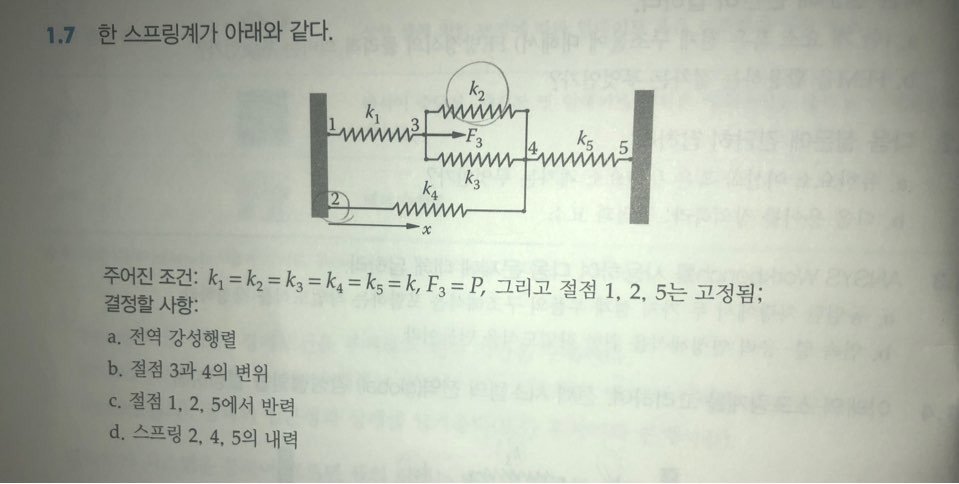```

Oh, and I would appreciate it if you could tell the answer using computer typing instead of handwriting.

`Because I don't know the cursive`

If it is difficult, I would appreciate it if you could respond in a convenient way.

1.7 한 스프링계가 아래와 같다. k2 ki -F3 Amino k4 K3 X 주어진 조건: k = k = kg = k 4 = ks = k, F = P, 그리고 절점 1, 2, 5는 고정됨; 결정할 사항: a. 전역 강성행렬 b. 절점 3과 4의 변위 C. 절점 1, 2, 5에서 반력 d. 스프링 2, 4, 5의 내력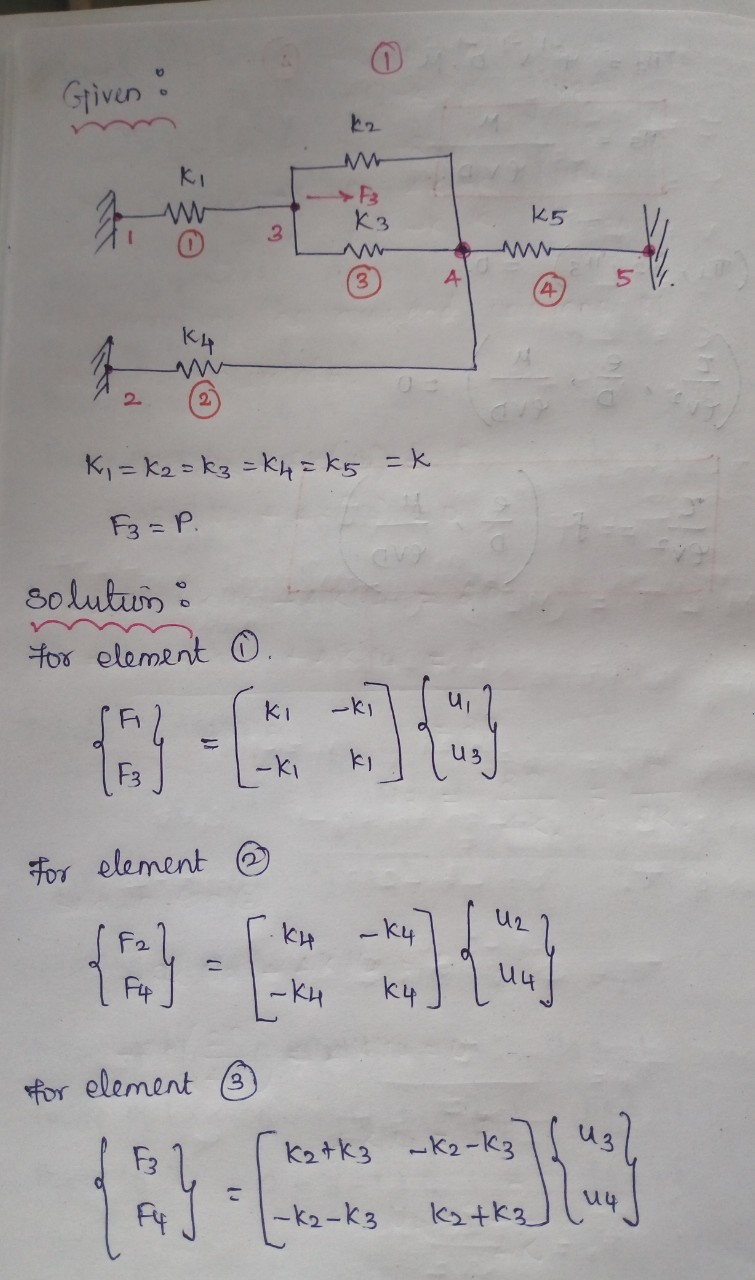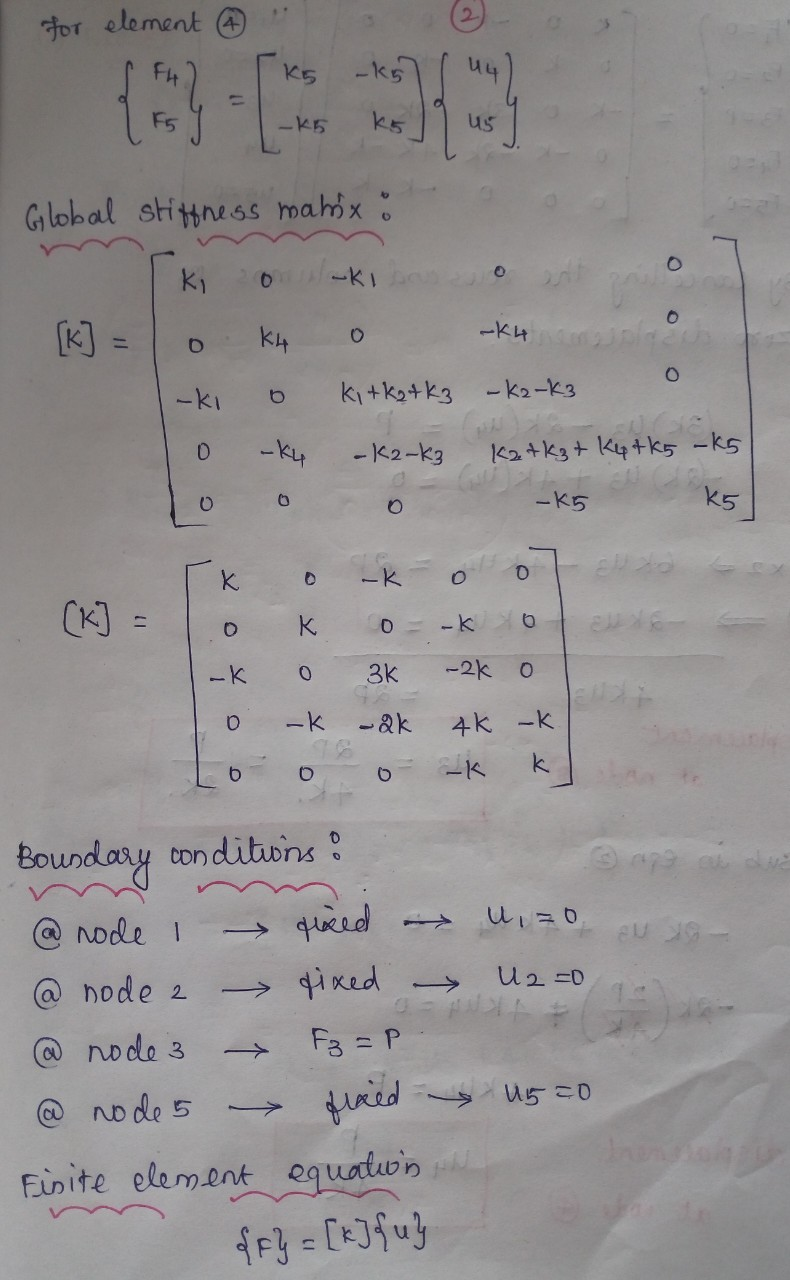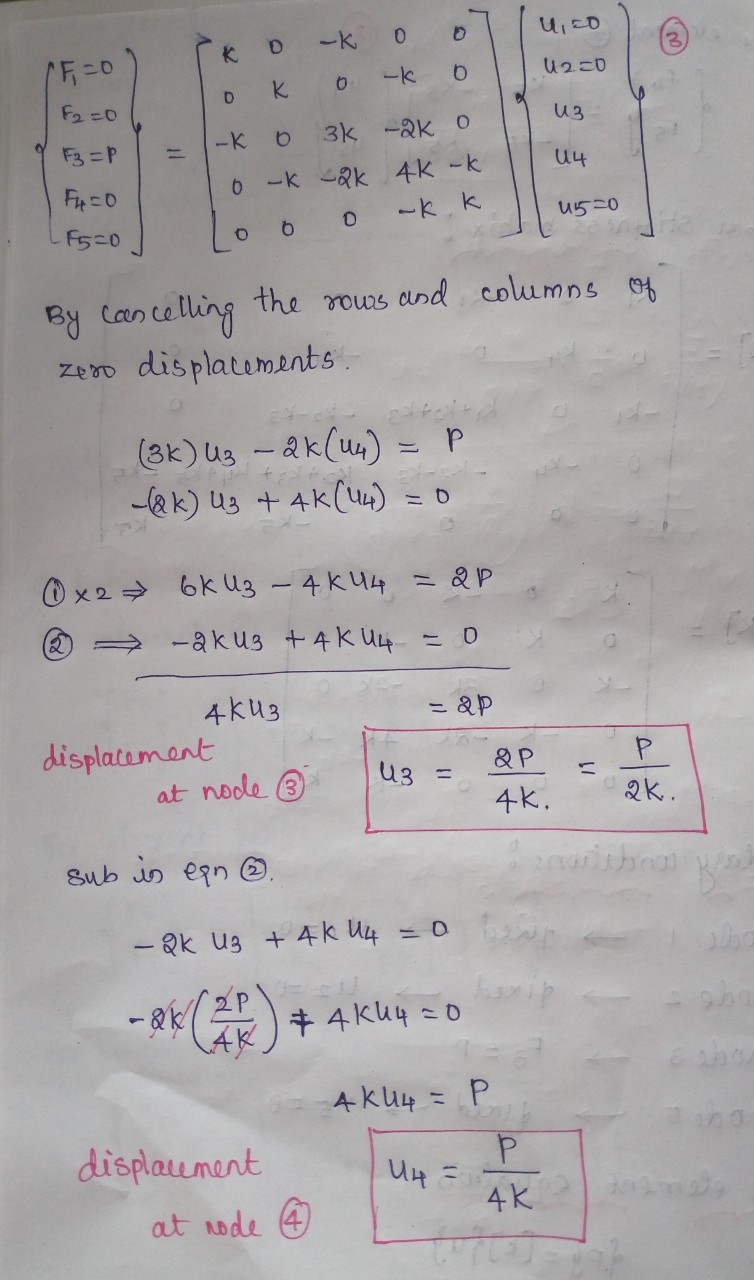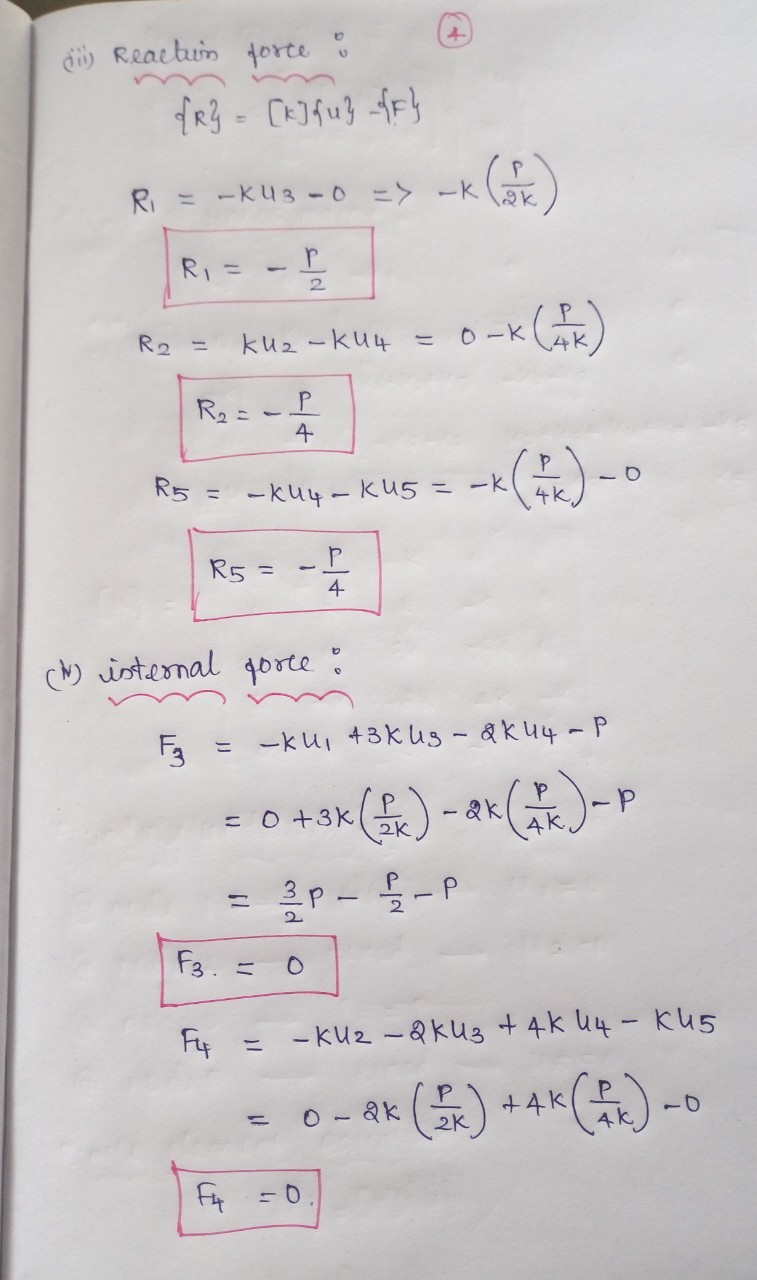#### Earn Coin

Coins can be redeemed for fabulous gifts.

Similar Homework Help Questions
• ### 2 k3 2 3 4 a. (10 points) Obtain the global stiffness matrix K using direct...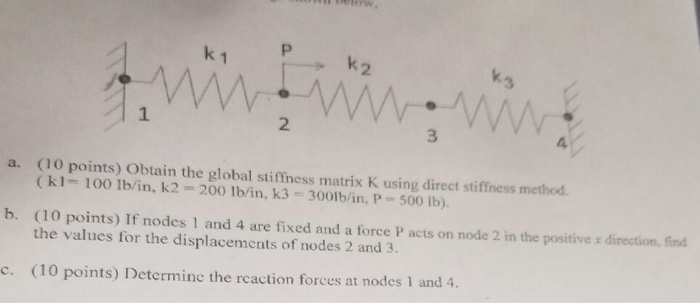2 k3 2 3 4 a. (10 points) Obtain the global stiffness matrix K using direct stiffness method (k1- 100 1b/in, k2 200 lb/in, k3 3001b/in, P-500 Ib). (10 points) If nodes 1 and 4 are fixed and a force P acts on node 2 in the positive x direction, fin the values for the displacements of nodes 2 and 3. b. c. (10 points) Deter odes 1 and 4

• ### Three rigid bodies (Nodes 2, 3, and 4) are connected by five springs as shown below....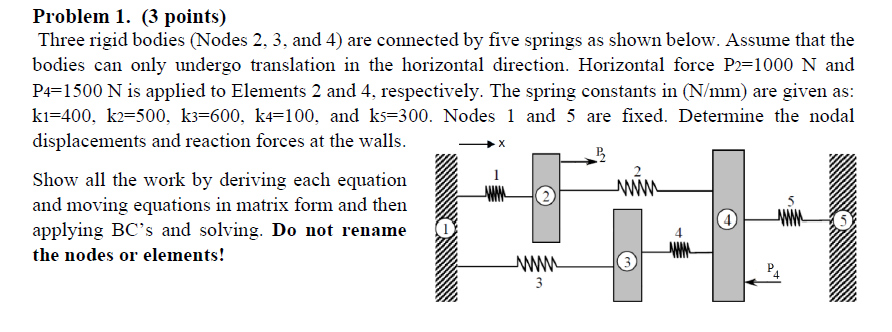Three rigid bodies (Nodes 2, 3, and 4) are connected by five springs as shown below. Assume that the bodies can only undergo translation in the horizontal direction. Horizontal force P2=1000 N and P4=1500 N is applied to Elements 2 and 4, respectively. The spring constants in (N/mm) are given as: k1=400, k2=500, k3=600, k4=100, and k5=300. Nodes 1 and 5 are fixed. Determine the nodal displacements and reaction forces at the walls. Problem 1. (3 points) Three rigid bodies...